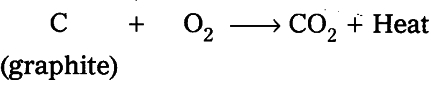# Calculate the mass and volume of oxygen

Calculate the mass and volume of oxygen required at STP to convert 2.4 kg of graphite into carbon dioxide.1 mole of graphite © = 12 gms
2.4 kg of graphite = 2400 / 12 = 200 moles
Mass of Oxygen required :
12 gms of graphite © requires 32 grams of oxygen
2.4 kg or 2400 grams of graphite requires = 2400 x 32 / 12 = 6400 gm or 6.4 kg of oxygen
Volume of oxygen required :
1 mole of carbon requires 1 mole of oxygen in 22.4 litres at STP 200 mole carbon
200 mole carbon requires = 200 x 22.4 = 4480 litres of {{O}_{2}} at STP